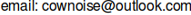# My Factor Collection -- $$a^n\pm1$$

### Introduction

This is a database of factors of numbers of the form $$a^n\pm1$$. It is mostly just the larger Brent-Montgomery-te Riele set which runs from base 2 to 9999 and exponent 1 to 9999. There is also a smaller set for $$a^n < 10^{255}$$ and 13 <= a <= 99.

### Check out these other active overlapping DB sites:

Cunningham Project $$a^n \pm 1; a \in \{2, 3, 5, 6, 7, 10, 11, 12\}$$
GIMPS Project $$2^p - 1$$
ECMNET Project ecm factors >= 60 digits; Cunningham Project factors
Studio Kamada $$10^n \pm 1$$
FactorDB all factors (but use judgement when x-posting factors as it may be considered spam.)"DON'T PANIC!" -- Hitchhiker's Guide To The Galaxy

Free DNS